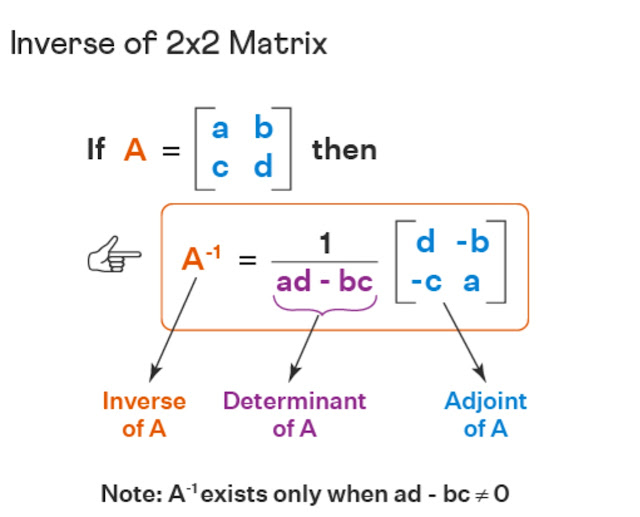# Matrices and Determinants Class 11 Mathematics Solutions | Exercise - 5.3## Chapter - 5  Matrices and Determinants

In linear algebra, Matrices and Determinants are used to linear equations by applying Cramer’s rule to a set of non-homogeneous equations which are in linear form. The ordered rectangular array of numbers used to express a linear equations are basically known as matrices.

Before we move towards the solution section of matrices and determinant, it's better for us to learn about the topics and subtopics involved in matrices and determinants chapter. So let's have a brief introduction of the matrices and determinants chapter.

### Introduction

As mentioned before, Matrices and determinants are used in linear algebra and it is defined a rectangular array of numbers, symbols, or expressions arranged in rows and columns whereas determinant is a number that is derived from the elements in a matrix and it is used to describe the properties of the matrix.

Matrices and determinants chapter helps students in understanding the concept of linear equations and their solutions. It will hwlp you to learn how you can make use of matrices in solving systems of linear equations. In this chapter, we will learn about different properties of matrices and determinants, which are essential in solving problems related to matrices and determinants.

If you want to solve all problems of this exercise then you should be well known about adjoint of matrix.  To understand it in simple language, the adjoint of a matrix is the transpose of its cofactor matrix and the cofactor matrix is obtained by taking the determinant of the minor matrix for each element of the original matrix. The adjoint matrix can be used to compute the inverse of a matrix, if it exists.

### Inverse of Matrix

In linear algebra, the inverse of a matrix is a matrix that, when multiplied with the original matrix, produces the identity matrix. It is denoted by A⁻¹, where A is the original matrix. The inverse of a matrix is used to solve linear equations. For a matrix to have its inverse the given matrix should be a square matrix and the determinant of that matrix should not be equal to zero.### Exercise - 5.3

In this PDF, you'll only find the solution of class 11 matrices and determinants chapter. It contains all the solutions of exercise- 5.3. If you want the solutions of other exercises then you'll find them above this PDF. Just click on the button and you'll reach your destination.

NoteScroll the PDF to view all Solution

You are not allowed to post this PDF in any website or social platform without permission.

## Is Class 11 Mathematics Guide Helpful For Student ?

I have published this Notes for helping students who can't solve difficult maths problems. Student should not fully depend on this note for completing all the exercises. If you totally depend on this note and simply copy as it is then it may affect your study.

Student should also use their own will power and try to solve problems themselves. You can use this mathematics guide PDF as a reference. You should check all the answers before copying because all the answers may not be correct. There may be some minor mistakes in the note, please consider those mistakes.

## How to secure good marks in Mathematics ?

As, you may know I'm also a student. Being a student is not so easy. You have to study different subjects simultaneously. From my point of view most of the student are weak in mathematics. You can take me as an example, I am also weak in mathematics. I also face problems while solving mathematics questions .

If you want to secure good marks in mathematics then you should practise them everyday. You should once revise all the exercise which are already taught in class. When you are solving maths problems, start from easy questions that you know already. If you do so then you won't get bored.

Maths is not only about practising, especially in grade 11 you to have the basic concept of the problem. When you get the main concept of the problem then you can easily any problems in which similar concept are applied.

When your teacher tries to make the concept clear by giving examples then all students tries to remember the same example but you should never do that. You can create your own formula which you won't forget later.

If you give proper time for your practise with proper technique then you can definitely score a good marks in your examination.

Disclaimer: This website is made for educational purposes. If you find any content that belongs to you then contact us through the contact form. We will remove that content from our as soon as possible.

If you have any queries then feel free to comment down.
No Comment